Next: 7.5 Theorems About Convergent Up: 7. Complex Sequences Previous: 7.3 Null Sequences   Index

# 7.4 Sums and Products of Null Sequences

7.27   Theorem (Sum theorem for null sequences.)Letbe complex null sequences and let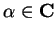. Then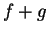,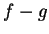, andare null sequences.

Scratchwork for: I want to find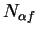so that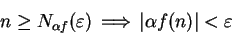i.e.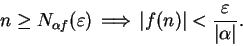This suggests that I take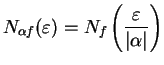.

Scratchwork for: I want to find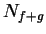so that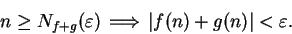Now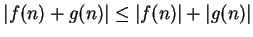, and I can make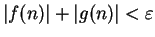by making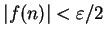and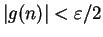. Hence I want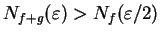and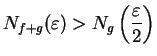. This suggests that I take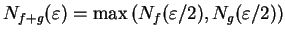.

Proof: Letbe null sequences, and let. Define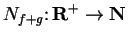by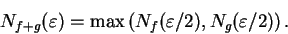Then for all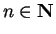,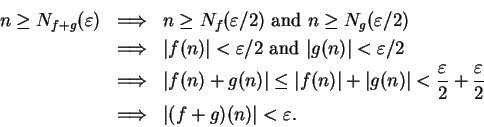Hence,is a precision function for, andis a null sequence.

If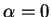thenis a null sequence. Suppose, and define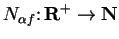by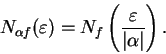Then for all,Henceis a precision function for, and henceis a null sequence. Since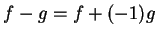it follows thatis a null sequence.7.28   Exercise (Product theorem for null sequences.) A Letbe complex null sequences. Prove that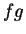is a null sequence.Next: 7.5 Theorems About Convergent Up: 7. Complex Sequences Previous: 7.3 Null Sequences   Index